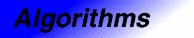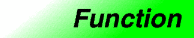# includesCategory: algorithms Component type: function

## Prototype

`Includes` is an overloaded name; there are actually two `includes` functions.

```template <class InputIterator1, class InputIterator2>
bool includes(InputIterator1 first1, InputIterator1 last1,
InputIterator2 first2, InputIterator2 last2);

template <class InputIterator1, class InputIterator2, class StrictWeakOrdering>
bool includes(InputIterator1 first1, InputIterator1 last1,
InputIterator2 first2, InputIterator2 last2,
StrictWeakOrdering comp);
```

## Description

`Includes` tests whether one sorted range includes another sorted range. That is, it returns `true` if and only if, for every element in `[first2, last2)`, an equivalent element  is also present in `[first1, last1)` . Both `[first1, last1)` and `[first2, last2)` must be sorted in ascending order.

The two versions of `includes` differ in how they define whether one element is less than another. The first version compares objects using `operator<`, and the second compares objects using the functors `comp`.

## Definition

Defined in the standard header algorithm, and in the nonstandard backward-compatibility header algo.h.

## Requirements on types

For the first version:

• `InputIterator1` is a model of InputIterator.
• `InputIterator2` is a model of InputIterator.
• `InputIterator1` and `InputIterator2` have the same value type.
• `InputIterator`'s value type is a model of LessThanComparable.
• The ordering on objects of `InputIterator1`'s value type is a strict weak ordering, as defined in the LessThanComparable requirements.

For the second version:

• `InputIterator1` is a model of InputIterator.
• `InputIterator2` is a model of InputIterator.
• `InputIterator1` and `InputIterator2` have the same value type.
• `StrictWeakOrdering` is a model of StrictWeakOrdering.
• `InputIterator1`'s value type is convertible to `StrictWeakOrdering`'s argument type.

## Preconditions

For the first version:

• `[first1, last1)` is a valid range.
• `[first2, last2)` is a valid range.
• `[first1, last1)` is ordered in ascending order according to `operator<`. That is, for every pair of iterators `i` and `j` in `[first1, last1)` such that `i` precedes `j`, `*j < *i` is `false`.
• `[first2, last2)` is ordered in ascending order according to `operator<`. That is, for every pair of iterators `i` and `j` in `[first2, last2)` such that `i` precedes `j`, `*j < *i` is `false`.

For the second version:

• `[first1, last1)` is a valid range.
• `[first2, last2)` is a valid range.
• `[first1, last1)` is ordered in ascending order according to `comp`. That is, for every pair of iterators `i` and `j` in `[first1, last1)` such that `i` precedes `j`, `comp(*j, *i)` is `false`.
• `[first2, last2)` is ordered in ascending order according to `comp`. That is, for every pair of iterators `i` and `j` in `[first2, last2)` such that `i` precedes `j`, `comp(*j, *i)` is `false`.

## Complexity

Linear. Zero comparisons if either `[first1, last1)` or `[first2, last2)` is an empty range, otherwise at most `2 * ((last1 - first1) + (last2 - first2)) - 1` comparisons.

## Example

```int A1[] = { 1, 2, 3, 4, 5, 6, 7 };
int A2[] = { 1, 4, 7 };
int A3[] = { 2, 7, 9 };
int A4[] = { 1, 1, 2, 3, 5, 8, 13, 21 };
int A5[] = { 1, 2, 13, 13 };
int A6[] = { 1, 1, 3, 21 };

const int N1 = sizeof(A1) / sizeof(int);
const int N2 = sizeof(A2) / sizeof(int);
const int N3 = sizeof(A3) / sizeof(int);
const int N4 = sizeof(A4) / sizeof(int);
const int N5 = sizeof(A5) / sizeof(int);
const int N6 = sizeof(A6) / sizeof(int);

cout << "A2 contained in A1: "
<< (includes(A1, A1 + N1, A2, A2 + N2) ? "true" : "false") << endl;
cout << "A3 contained in A1: "
<< (includes(A1, A1 + N2, A3, A3 + N3) ? "true" : "false") << endl;
cout << "A5 contained in A4: "
<< (includes(A4, A4 + N4, A5, A5 + N5) ? "true" : "false") << endl;
cout << "A6 contained in A4: "
<< (includes(A4, A4 + N4, A6, A6 + N6) ? "true" : "false") << endl;
```

The output is:

```A2 contained in A1: true
A3 contained in A1: false
A5 contained in A4: false
A6 contained in A4: true
```